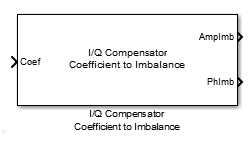Main Content

# I/Q Compensator Coefficient to Imbalance

Convert compensator coefficient into amplitude and phase imbalance

## Library

RF Impairments Correction

•## Description

The I/Q Compensator Coefficient to Imbalance block converts a compensator coefficient into its equivalent amplitude and phase imbalance.

This block has a single input port, which accepts a complex coefficient or a vector of coefficients. There are amplitude and phase imbalance output ports both of which are real. The amplitude imbalance is expressed in dB while the phase imbalance is expressed in degrees.

## Algorithms

See the `iqcoef2imbal` function reference page for more information on the inputs, outputs, and algorithms.

## Supported Data Types

PortSupported Data Types
Compensator Coefficient
• Double-precision, complex floating point

• Single-precision, complex floating point

Amplitude Imbalance (dB)
• Double-precision floating point

• Single-precision floating point

Phase Imbalance (deg)
• Double-precision floating point

• Single-precision floating point

## See Also

### Functions

Introduced in R2014b

## Support

#### Bridging Wireless Communications Design and Testing with MATLAB

Download white paper